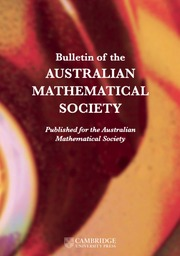Home
Hostname: page-component-56f9d74cfd-b2czv Total loading time: 0.307 Render date: 2022-06-28T06:14:17.417Z Has data issue: true Feature Flags: { "shouldUseShareProductTool": true, "shouldUseHypothesis": true, "isUnsiloEnabled": true, "useRatesEcommerce": false, "useNewApi": true }Bulletin of the Australian Mathematical Society

# ON THE DIVISIBILITY AMONG POWER LCM MATRICES ON GCD-CLOSED SETS

Published online by Cambridge University Press:  19 May 2022

*

## Abstract

Let$a,b$ and n be positive integers and let$S=\{x_1, \ldots , x_n\}$ be a set of n distinct positive integers. For${x\in S}$ , define$G_{S}(x)=\{d\in S: d<x, \,d\mid x \ \mathrm {and} \ (d\mid y\mid x, y\in S)\Rightarrow y\in \{d,x\}\}$ . Denote by$[S^a]$ the$n\times n$ matrix having the ath power of the least common multiple of$x_i$ and$x_j$ as its$(i,j)$ -entry. We show that the bth power matrix$[S^b]$ is divisible by the ath power matrix$[S^a]$ if$a\mid b$ and S is gcd closed (that is,$\gcd (x_i, x_j)\in S$ for all integers i and j with$1\le i, j\le n$ ) and$\max _{x\in S} \{|G_S (x)|\}=1$ . This confirms a conjecture of Shaofang Hong [‘Divisibility properties of power GCD matrices and power LCM matrices’, Linear Algebra Appl.428 (2008), 1001–1008].

## MSC classification

Type
Research Article
© The Author(s), 2022. Published by Cambridge University Press on behalf of Australian Mathematical Publishing Association Inc.

## Access options

Get access to the full version of this content by using one of the access options below. (Log in options will check for institutional or personal access. Content may require purchase if you do not have access.)

## References

Altinisik, E., Yildiz, M. and Keskin, A., ‘Non-divisibility of LCM matrices by GCD matrices on gcd-closed sets’, Linear Algebra Appl. 516 (2017), 4768.CrossRefGoogle Scholar
Bege, A., ‘Generalized LCM matrices’, Publ. Math. Debrecen 79 (2011), 309315.CrossRefGoogle Scholar
Beslin, S. and Ligh, S., ‘Another generalization of Smith’s determinant’, Bull. Aust. Math. Soc. 40 (1989), 413415.CrossRefGoogle Scholar
Bourque, K. and Ligh, S., ‘On GCD and LCM matrices’, Linear Algebra Appl. 174 (1992), 6574.CrossRefGoogle Scholar
Bourque, K. and Ligh, S., ‘Matrices associated with arithmetical functions’, Linear Multilinear Algebra 34 (1993), 261267.CrossRefGoogle Scholar
Chen, L., Feng, Y. L., Hong, S. F. and Qiu, M., ‘On the divisibility of matrices associated with multiplicative functions’, Publ. Math. Debrecen 100 (2022), 323335.CrossRefGoogle Scholar
Feng, W. D., Hong, S. F. and Zhao, J. R., ‘Divisibility properties of power LCM matrices by power GCD matrices on gcd-closed sets’, Discrete Math. 309 (2009), 26272639.CrossRefGoogle Scholar
Hong, S. A., Hu, S. N. and Lin, Z. B., ‘On a certain arithmetical determinant’, Acta Math. Hungar. 150 (2016), 372382.CrossRefGoogle Scholar
Hong, S. F., ‘On the Bourque–Ligh conjecture of least common multiple matrices’, J. Algebra 218 (1999), 216228.CrossRefGoogle Scholar
Hong, S. F., ‘On the factorization of LCM matrices on gcd-closed sets’, Linear Algebra Appl. 345 (2002), 225233.CrossRefGoogle Scholar
Hong, S. F., ‘Notes on power LCM matrices’, Acta Arith. 111 (2004), 165177.CrossRefGoogle Scholar
Hong, S. F., ‘Divisibility properties of power GCD matrices and power LCM matrices’, Linear Algebra Appl. 428 (2008), 10011008.CrossRefGoogle Scholar
Hong, S. F., Zhao, J. R. and Yin, Y. Z., ‘Divisibility properties of Smith matrices’, Acta Arith. 132 (2008), 161175.CrossRefGoogle Scholar
Korkee, I. and Haukkanen, P., ‘On the divisibility of meet and join matrices’, Linear Algebra Appl. 429 (2008), 19291943.CrossRefGoogle Scholar
Smith, H. J. S., ‘On the value of a certain arithmetical determinant’, Proc. Lond. Math. Soc. (3) 7 (1875), 208212.CrossRefGoogle Scholar
Tan, Q. R. and Li, M., ‘Divisibility among power GCD matrices and among power LCM matrices on finitely many coprime divisor chains’, Linear Algebra Appl. 438 (2013), 14541466.CrossRefGoogle Scholar
Zhao, J. R., ‘Divisibility of power LCM matrices by power GCD matrices on gcd-closed sets’, Linear Multilinear Algebra 62 (2014), 735748.CrossRefGoogle Scholar
Zhao, J. R., Chen, L. and Hong, S. F., ‘Gcd-closed sets and divisibility of Smith matrices’, J. Combin. Theory Ser. A 188 (2022), Article no. 105581, 23 pages.CrossRefGoogle Scholar
Zhu, G. Y., ‘On the divisibility among power GCD and power LCM matrices on gcd-closed sets’, Int. J. Number Theory, to appear.Google Scholar
Zhu, G. Y., ‘On a certain determinant for a U.F.D.’, Colloq. Math., to appear.Google Scholar
Zhu, G. Y., Cheng, K. M. and Zhao, W., ‘Notes on Hong’s conjecture on nonsingularity of power LCM matrices’, AIMS Math. 7 (2022), 1027610285.CrossRefGoogle Scholar

# Save article to Kindle

Note you can select to save to either the @free.kindle.com or @kindle.com variations. ‘@free.kindle.com’ emails are free but can only be saved to your device when it is connected to wi-fi. ‘@kindle.com’ emails can be delivered even when you are not connected to wi-fi, but note that service fees apply.

Find out more about the Kindle Personal Document Service.

ON THE DIVISIBILITY AMONG POWER LCM MATRICES ON GCD-CLOSED SETS
Available formats
×

# Save article to Dropbox

To save this article to your Dropbox account, please select one or more formats and confirm that you agree to abide by our usage policies. If this is the first time you used this feature, you will be asked to authorise Cambridge Core to connect with your Dropbox account. Find out more about saving content to Dropbox.

ON THE DIVISIBILITY AMONG POWER LCM MATRICES ON GCD-CLOSED SETS
Available formats
×

# Save article to Google Drive

To save this article to your Google Drive account, please select one or more formats and confirm that you agree to abide by our usage policies. If this is the first time you used this feature, you will be asked to authorise Cambridge Core to connect with your Google Drive account. Find out more about saving content to Google Drive.

ON THE DIVISIBILITY AMONG POWER LCM MATRICES ON GCD-CLOSED SETS
Available formats
×
×